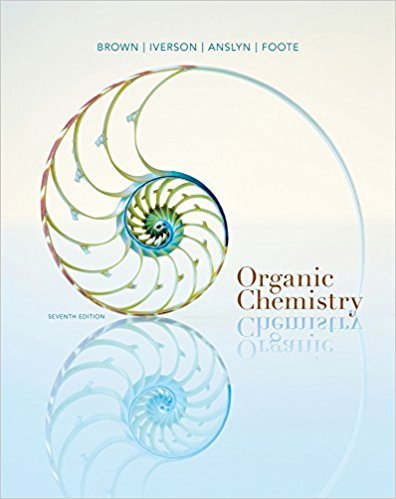×
Get Full Access to Organic Chemistry - 7 Edition - Chapter 14 - Problem 14.2
Get Full Access to Organic Chemistry - 7 Edition - Chapter 14 - Problem 14.2

×

# Propose a structural formula for the cation at m/z 41 observed in the massspectrum ofISBN: 9781133952848 483

## Solution for problem 14.2 Chapter 14

Organic Chemistry | 7th Edition

• Textbook Solutions
• 2901 Step-by-step solutions solved by professors and subject experts
• Get 24/7 help from StudySoup virtual teaching assistantsOrganic Chemistry | 7th Edition

4 5 1 430 Reviews
13
1
Problem 14.2

Propose a structural formula for the cation at m/z 41 observed in the massspectrum of methylcyclopentane.

Step-by-Step Solution:
Step 1 of 3

Ligands o Monodentate  Only coordinates to the metal at one spot on molecule o Bidentate & Polydentate  Can coordinate to the metal at multiple spots  Typically larger molecules  i.e. Ethylenediamine (en) below  Formation Constants o Complex with larger formation constant forms more favorably o K fiffer because of Gibbs Free Energy  Chelate Effect (kee-late)  Larger K fave larger change in entropy  Chelating Agents o Remove or separate metal ions from a solution o i.e. EDTA removes poisonous trace metals in food  Energy in Coordinate Chemistry o Ligand’s electrons are attracted to metal cation  Increases energy of the d-orbitals o Crystal Field Splitting  Difference in energy among d-orbitals based on ligand geometry  Used to explain many observations about coordinate chemistry, such as color and magnetism  Octohedral Geometry  Ligands are along x, y, z axes  Raises energy of d z2and d x -yorbitals the most  Raises energy of other d orbitals less  Tetrahedral Geometry  Ligands come between axes 2 2 2  Raises energy of d z and d x -y orbitals less  Raises energy of other d orbitals th

Step 2 of 3

Step 3 of 3

##### ISBN: 9781133952848

This full solution covers the following key subjects: . This expansive textbook survival guide covers 24 chapters, and 1131 solutions. Since the solution to 14.2 from 14 chapter was answered, more than 347 students have viewed the full step-by-step answer. The full step-by-step solution to problem: 14.2 from chapter: 14 was answered by , our top Chemistry solution expert on 03/16/18, 05:02PM. This textbook survival guide was created for the textbook: Organic Chemistry, edition: 7. The answer to “Propose a structural formula for the cation at m/z 41 observed in the massspectrum of methylcyclopentane.” is broken down into a number of easy to follow steps, and 16 words. Organic Chemistry was written by and is associated to the ISBN: 9781133952848.

## Discover and learn what students are asking

Chemistry: The Central Science : Liquids and Intermolecular Forces
?The phase diagram of a hypothetical substance is (a) Estimate the norm

Statistics: Informed Decisions Using Data : Estimating a Population Standard Deviation
?5. 90% confidence, n = 20

Statistics: Informed Decisions Using Data : Comparing Three or More Means (One-Way Analysis of Variance)
?In Problems 7 and 8, fill in the ANOVA table.

Statistics: Informed Decisions Using Data : Inference about the Difference between Two Medians: Dependent Samples
?In Problems 3–10, use the Wilcoxon matched-pairs signedranks test to test the given hypotheses at the a = 0.05 level of significance. The dependent sa

Chemistry: The Central Science : The Chemistry of Life: Organic and Biological Chemistry
?Give the the name or condensed structural formula, as appropriate: (c) 2-me

Unlock Textbook Solution